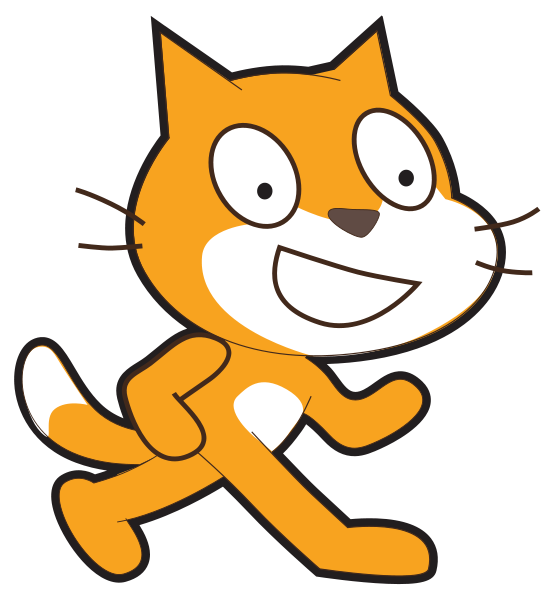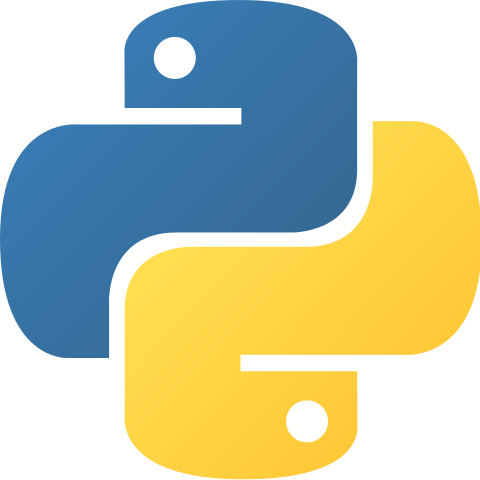# Display the binary cards needed to represent a given number of dots (all in one line)

Challenge Level: Growing experience

Learning outcomesStudents will be able to:

## Requirement:

Write a program that asks the user to enter a number of dots less than or equal to 31 as the input and displays which of the 5 cards should be showing as the output all in one line.

## Testing examples:

Your program should display the outputs shown in this table for the given inputs provided;

Input Output
15 8, 4, 2, 1,
1 1,
8 8,
31 16, 8, 4, 2, 1,
32 Please choose a number less than or equal to 31.

## Languages

Scratch#### What it should look like

Click on the green flag, enter the inputs provided in the “testing examples” to see the expected output of your program.

Recommended blocks
when green flag clicked

ask [Please enter a number of dots less than or equal to 31:] and wait
if <<(number of dots) > > or <(number of dots) = >> then
end

if <<(number of dots) > > or <(number of dots) = >> then
end

if <<(number of dots) > > or <(number of dots) = >> then
end

if <<(number of dots) > > or <(number of dots) = >> then
end

if <<(number of dots) > > or <(number of dots) = >> then
end

if <<(number of dots) < > or <(number of dots) = >> then
else
end
set [number of dots v] to (answer)

set [cards v] to []

set [cards v] to (join (cards) [16, ])

set [number of dots v] to ((number of dots) - (16))

set [cards v] to (join (cards) [8, ])

set [number of dots v] to ((number of dots) - (8))

set [cards v] to (join (cards) [4, ])

set [number of dots v] to ((number of dots) - (4))

set [cards v] to (join (cards) [2, ])

set [number of dots v] to ((number of dots) - (2))

set [cards v] to (join (cards) [1, ])

set [number of dots v] to ((number of dots) - (1))
say (cards)

say [Please choose a number less than or equal to 31.]
Hints
• Make a variable called “number of dots” and set its value to the input entered by the end user. Make another variable called “cards”. Below is the algorithm to help you program this:
If “number of dots” less than or equal to 31
If “number of dots” greater than or equal to 16
Subtract 16 from “number of dots” and add “16, ” to string variable “cards”
If “number of dots” greater than or equal to 8
Subtract 8 from “number of dots” and add “8, ” to string variable “cards”
If “number of dots” greater than or equal to 4
Subtract 4 from “number of dots” and add “4, ” to string variable “cards”
If “number of dots” greater than or equal to 2
Subtract 2 from “number of dots” and add “2, ” to string variable “cards”
If “number of dots” greater than or equal to 1
Subtract 1 from “number of dots” and add “1, ” to string variable “cards”
Display the value of “cards”
else
Ask the end user to enter a number less than or equal to “31”
• The if <> then block checks if the condition is true and then runs the blocks inside of the IF block. In this challenge you need to use if <> then blocks to check if the “number of dots” is greater than or equal to 16 (use the <> or <> block under “Operators”). If it is, display card “16” and subtract 16 from your number and do the same with the rest of the cards.
• You can also use the IF THEN ELSE block (see image below) if you need to run a set of blocks if the condition is not true.
if <> then
else
end
• In the extra challenge you need to ask the user to enter a number less than or equal to 31 if the input which was entered was greater than 31.
• Test your program with some values on the boundaries (i.e. 32, 31, 16, 8, 4, 2, 1)
Python## Extra Challenge

Now try removing the last , from the end of your output.